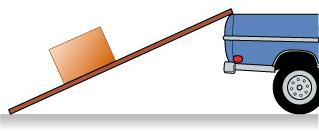# Problem: A large crate filled with physics laboratory equipment must be moved up an incline onto a truck.1. The crate is at rest on the incline. What can you say about the force of friction acting on the crate?A. the frictional force points up the inclineB. the frictional force points down the inclineC. the frictional force is zero2. A physicist attempts to push the crate up the incline. The physicist senses that if he applies slightly more force the crate will move up the incline but cannot muster enough strength to get the motion started. What can you say now about the force of friction acting on the crate?A. the frictional force points up the inclineB. the frictional force points down the inclineC. the frictional force is zero3. The first physicist gets a second physicist to help. They both push on the crate, parallel to the surface of the incline, and it moves at constant speed up the incline. How does the force exerted by the two physicists on the crate compare with the force of friction on the crate?A. force of 2 physicists &lt; force of frictionB. force of 2 physicists = force of frictionC. force of 2 physicists &gt; force of friction

###### FREE Expert Solution

1.

Suppose there is no friction, the box will move downward due to gravity.

Frictional force must act in upward direction of the incline.

Because frictional force must act in the upward direction of the incline, the frictional force cannot point down in the incline and cannot be zero.

83% (191 ratings)###### Problem Details

A large crate filled with physics laboratory equipment must be moved up an incline onto a truck.1. The crate is at rest on the incline. What can you say about the force of friction acting on the crate?

A. the frictional force points up the incline

B. the frictional force points down the incline

C. the frictional force is zero

2. A physicist attempts to push the crate up the incline. The physicist senses that if he applies slightly more force the crate will move up the incline but cannot muster enough strength to get the motion started. What can you say now about the force of friction acting on the crate?

A. the frictional force points up the incline

B. the frictional force points down the incline

C. the frictional force is zero

3. The first physicist gets a second physicist to help. They both push on the crate, parallel to the surface of the incline, and it moves at constant speed up the incline. How does the force exerted by the two physicists on the crate compare with the force of friction on the crate?

A. force of 2 physicists < force of friction

B. force of 2 physicists = force of friction

C. force of 2 physicists > force of friction